# ADDITION OF VECTORS, INTERMEDIATE FIRST YEAR MATHEMATICS 1A PROBLEMS WITH SOLUTIONS

Intermediate mathematics first year 1A and 1B some chapters solutions. The solutions are very easy to understand.

First year 1A : Mathematical induction, functions, addition of vectors, trigonometric ratios upto transformations, trigonometric equations, hyperbolic functions, inverse trigonometric functions and properties of triangles.

First year 1B : Locus, transformation of axes and three dimensional coordinates, the straight line, pair of straight lines, the planes and applications of derivatives.

Observe the given solutions and try them in your own method

You can also see the text book solution for Addition of Vectors

Exercise 4(a)

# JUNIOR INTER MATHS ALGEBRA VECTORS

You can see the solutions for junior inter maths 1A

3. Matrices

Junior inter 1B solutions of junior inter 1B for examination purpose

1Locus

Straight lines sa

Straight lines la

12. Rate measure

You can see the solutions for text book Maths 1A

Functions

Exercise 1(a)

Exercise 1(b)

Exercise 1(c)

Mathematical Induction

Exercise 2(a)

Matrices

Exercise 3(a)

Exercise 3(b)

Exercise 3(c)

Exercise 3d)

Exercise 3(e)

Exercise 3(f)

Exercise 3(g)

Exercise 3(h)

Exercise 3(i)

Exercise 4(a)

Exercise 4(b)

Product of Vectors

Exercise 5(a)

Exercise 5(b)

Exercise 5(c)

Trigonometric Ratios up to Transformations

Exercise 6(a)

Exercise 6(b)

Exercise 6(c)

Exercise 6(d)

Exercise 6(e)

Exercise 6(f)

Trigonometric Equations

Exercise 7(a)

Inverse Trigonometric Equations

Exercise 8(a)

Hyperbolic Functions

Exercise 9(a)

Properties of Triangles

Exercise 10(a)

Exercise 10(b)

Maths 1B solutions for

Locus

Exercise 1(a)

Transformation of Axes

Exercise 2(a)

Three Dimensional Coordinates

Exercise 5(a)

Exercise 5(b)

Direction Cosines and Direction Ratios

Exercise 6(a)

The Plane

Exercise 7(a)

Limits and Continuity

Exercise 8(a)

Exercise 8(b)

Exercise 8(d)

Exercise 8(e)

M

### Addition of vectors solutions

Very short answer questions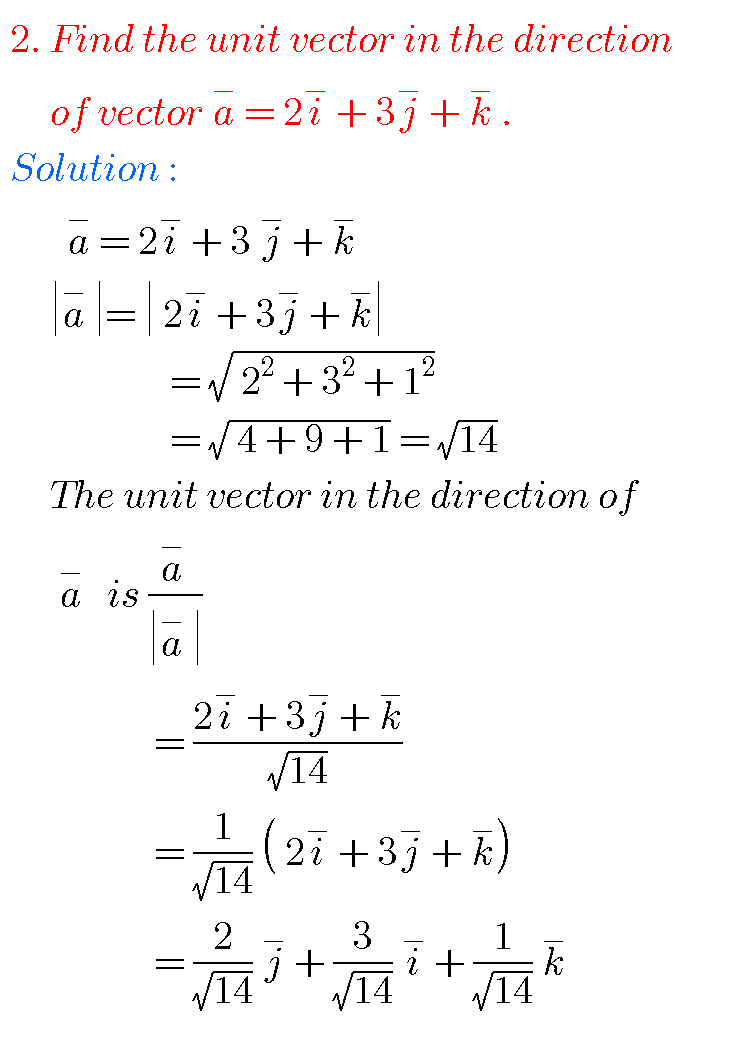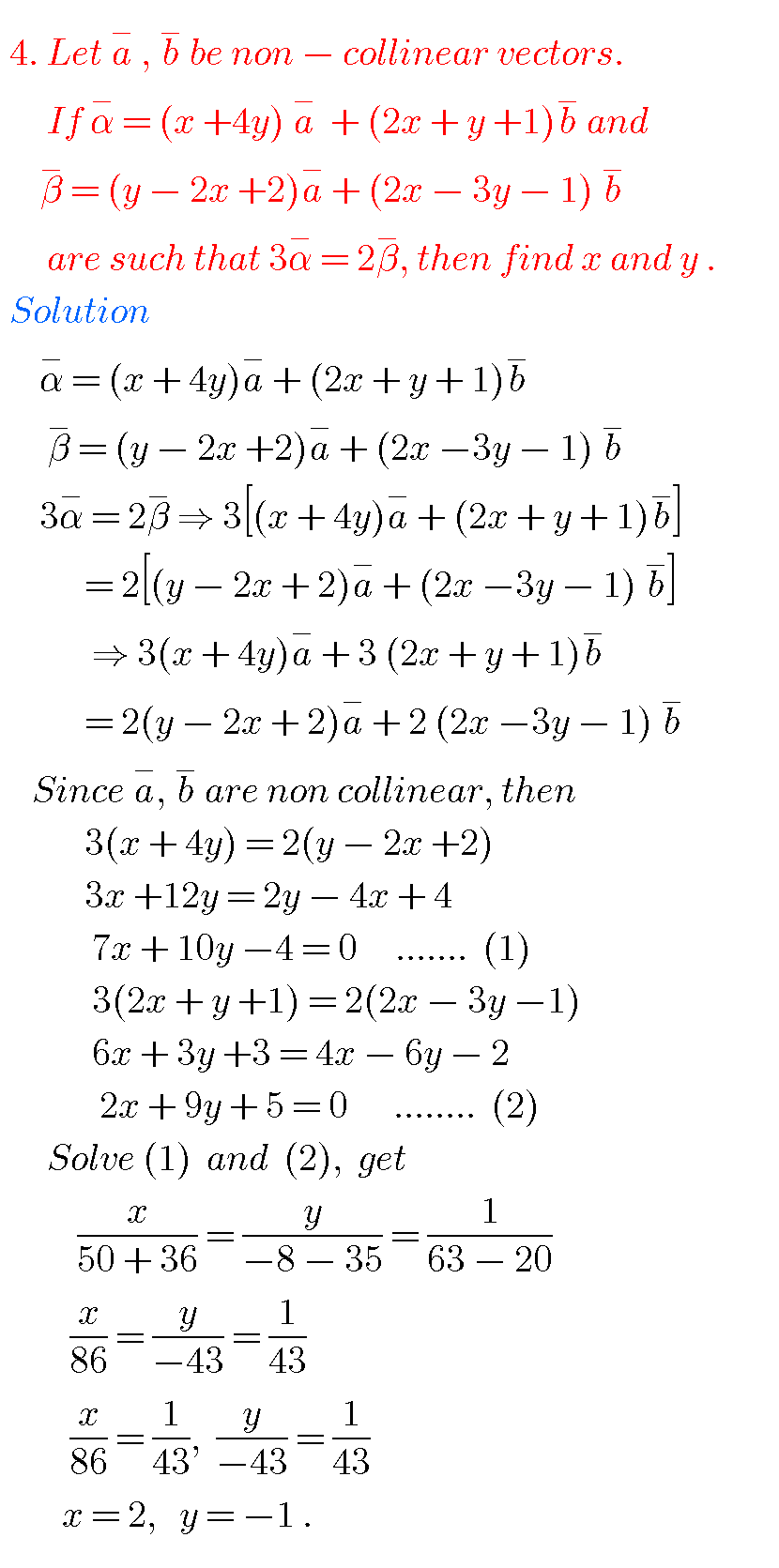##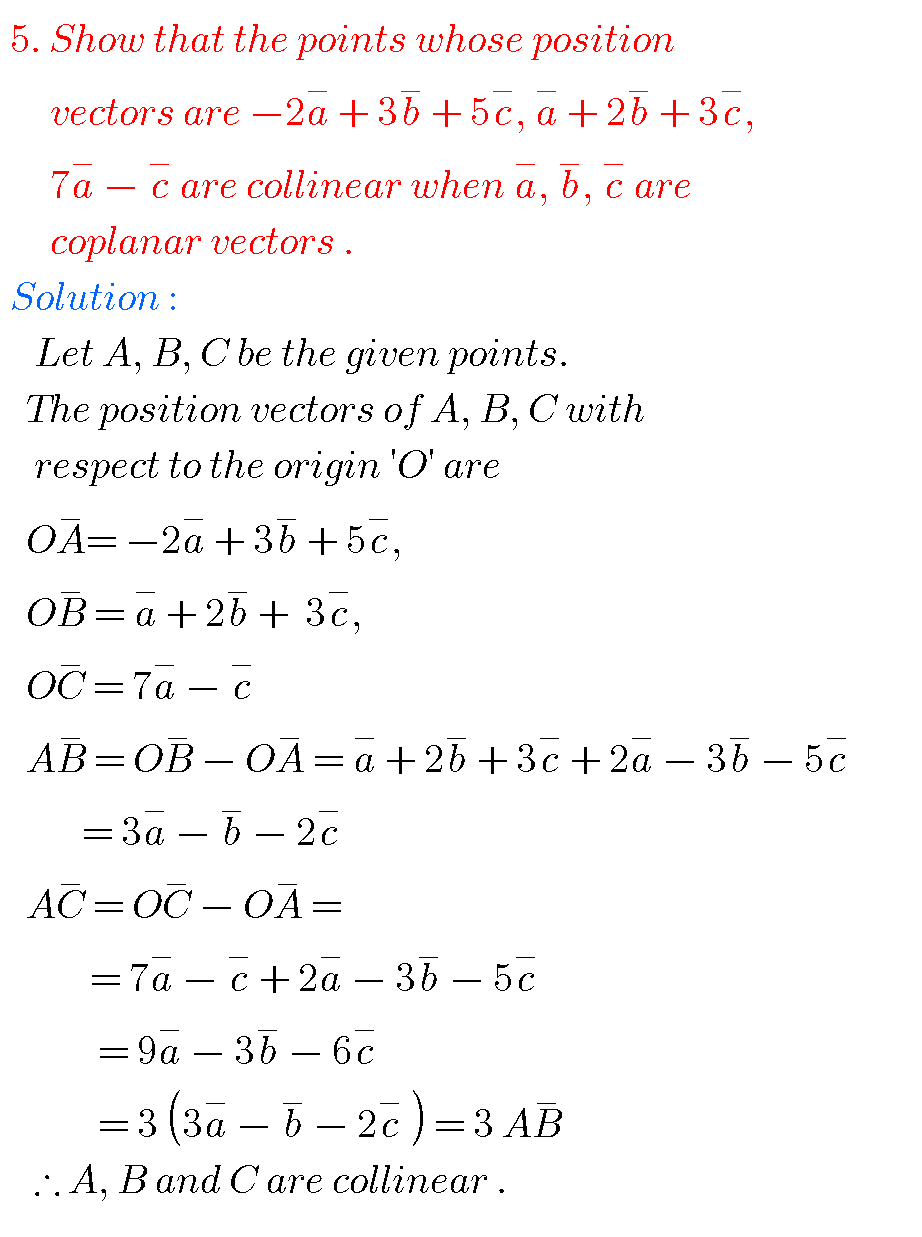##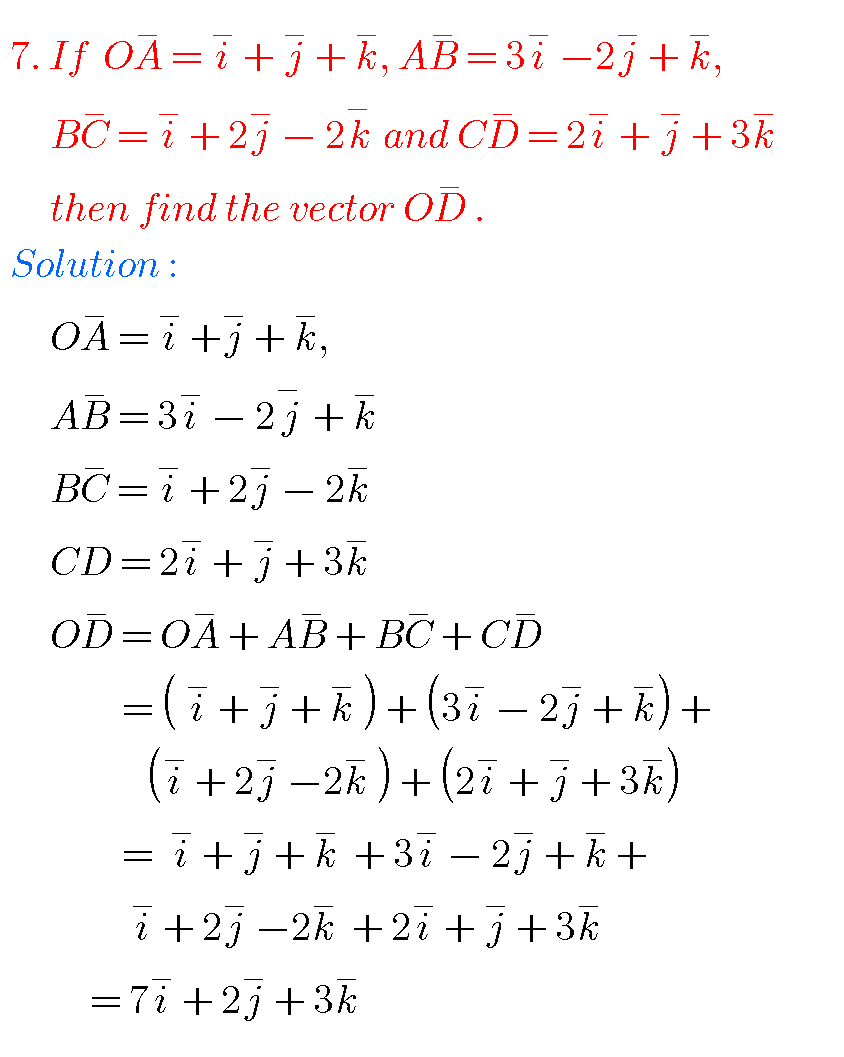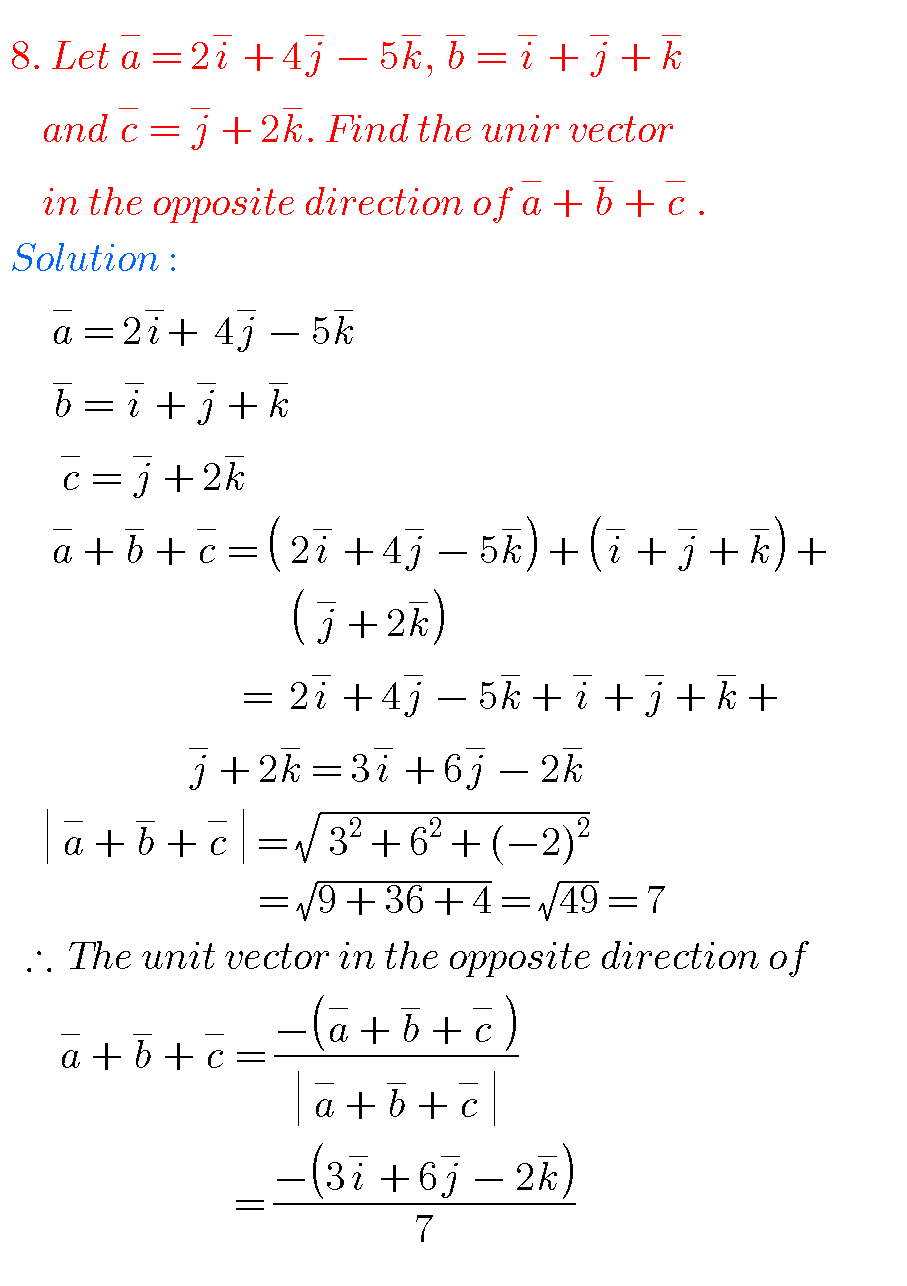## JUNIOR INTER MATHS VECTOR ALGEBRANote :

Observe the solutions and try them in your own methods.

You can also see the solutions of

1. Functions

2. Mathematical induction

3. Trigonometric ratios upto transformations 1

4. Trigonometric ratios upto transformations 2

5. Trigonometric equations

6. Inverse trigonometric functions

7. Hyperbolic functions

8. Properties of triangles

You can also see

Ncert solutions for maths class 6 some chapters

Ncert solutions for maths class 7 some chapters

Ncert solutions for maths class 8 some chapters

Ncert solutions for class 8 chapter 12

Inter maths 1a solutions

Inter maths trigonometry solutions

Maths real numbers solutions class 10

You can see solutions for Inter Maths IIB

1. Circle

3. Parabola

4. Ellipse

You can also see solutions for Inter Maths IIA

For examination purpose you can see

Complex numbers

De Moivre’ s Theorem